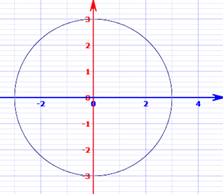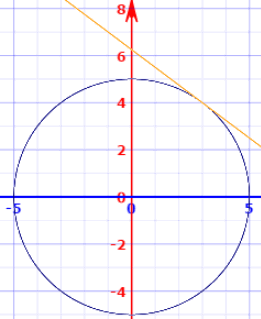# Implicit Differentiation

Finding the derivative when you can’t solve for yYou may like to read Introduction to Derivatives and Derivative Rules first.

## Implicit vs Explicit

A function can be explicit or implicit:

Explicit: "y = some function of x". When we know x we can calculate y directly.

Implicit: "some function of y and x equals something else". Knowing x does not lead directly to y.

### Example: A Circle

 Explicit Form Implicit Form y = ± √ (r2 − x2) x2 + y2 = r2 In this form, y is expressed as a function of x. In this form, the function is expressed in terms of both y and x.The graph of x2 + y2 = 32

## How to do Implicit Differentiation

• Differentiate with respect to x
• Collect all the dy dx on one side
• Solve for dy dx

### Example: x2 + y2 = r2

Differentiate with respect to x:

d dx (x2) + d dx (y2) = d dx (r2)

Let's solve each term:

Use the Power Rule: d dx (x2) = 2x
Use the Chain Rule (explained below): d dx (y2) = 2y dy dx
r2 is a constant, so its derivative is 0: d dx (r2) = 0

Which gives us:

2x + 2y dy dx = 0

Collect all the dy dx on one side

y dy dx = −x

Solve for dy dx :

dy dx = −x y

### The Chain Rule Using dy dx

Let's look more closely at how d dx (y2) becomes 2y dy dx

The Chain Rule says:

du dx = du dy dy dx

Substitute in u = y2:

d dx (y2) = d dy (y2) dy dx

And then:

d dx (y2) = 2y dy dx

### Basically, all we did was differentiate with respect to y and multiply by dy dx

Another common notation is to use to mean d dx

### The Chain Rule Using ’

The Chain Rule can also be written using notation:

f(g(x))’ = f’(g(x))g’(x)

g(x) is our function "y", so:

f(y)’ = f’(y)y’

f(y) = y2, so f(y) = 2y:

f(y)’ = 2yy’

or alternatively: f(y)’ = 2y dy dx

## Explicit

Let's also find the derivative using the explicit form of the equation.

• To solve this explicitly, we can solve the equation for y
• Then differentiate
• Then substitute the equation for y again

### Example: x2 + y2 = r2

Subtract x2 from both sides:y2 = r2 − x2
Square root:y = ±√(r2 − x2
Let's do just the positive: y = √(r2 − x2
As a power: y = (r2 − x2)½
Derivative (Chain Rule):y =½(r2 − x2)−½(2x)
Simplify:y = −x(r2 − x2)−½
Simplify more:y = −x (r2 − x2)½
Now, because y = (r2 − x2)½y = −x/y

We get the same result this way!

You can try taking the derivative of the negative term yourself.

### Chain Rule Again!

Yes, we used the Chain Rule again. Like this (note different letters, but same rule):

dy dx = dy df df dx

Substitute in f = (r2 − x2):

d dx (f½) = d df (f½) d dx (r2 − x2)

Derivatives:

d dx (f½) = ½(f−½) (−2x)

And substitute back f = (r2 − x2):

d dx (r2 − x2)½ = ½((r2 − x2)−½) (−2x)

And we simplified from there.

## Using The Derivative

OK, so why find the derivative y’ = −x/y ?

Well, for example, we can find the slope of a tangent line.

### Example: what is the slope of a circle centered at the origin with a radius of 5 at the point (3, 4)?No problem, just substitute it into our equation:

dy dx = −x/y

dy dx = −3/4

And for bonus, the equation for the tangent line is:

y = −3/4 x + 25/4

## Another Example

Sometimes the implicit way works where the explicit way is hard or impossible.

### Example: 10x4 − 18xy2 + 10y3 = 48

How do we solve for y? We don't have to!

• First, differentiate with respect to x (use the Product Rule for the xy2 term).
• Then move all dy/dx terms to the left side.
• Solve for dy/dx

Like this:

Derivative:10 (4x3) − 18(x(2y dy dx ) + y2) + 10(3y2 dy dx ) = 0

(the middle term is explained
in "Product Rule" below)

Simplify:40x3 − 36xy dy dx − 18y2 + 30y2 dy dx = 0
dy dx on left:−36xy dy dx + 30y2dy dx = −40x3 + 18y2
Simplify :(30y2−36xy) dy dx = 18y2 − 40x3
Simplify :3(5y2−6xy) dy dx = 9y2 − 20x3

And we get:

dydx = 9y2 − 20x33(5y2 − 6xy)

### Product Rule

For the middle term we used the Product Rule: (fg)’ = f g’ + f’ g

(xy2)’ = x(y2)’ + (x)’y2
= x(2y dy dx ) + y2

Because (y2)’  = 2y dy dx (we worked that out in a previous example)

Oh, and dxdx = 1, in other words x’ = 1

## Inverse Functions

Implicit differentiation can help us solve inverse functions.

The general pattern is:

• Start with the inverse equation in explicit form. Example: y = sin−1(x)
• Rewrite it in non-inverse mode: Example: x = sin(y)
• Differentiate this function with respect to x on both sides.
• Solve for dy/dx

As a final step we can try to simplify more by substituting the original equation.

An example will help:

### Example: the inverse sine function y = sin−1(x)

In non−inverse mode:x = sin(y)
Derivative: d dx (x) = d dx sin(y)
1 = cos(y) dy dx
Put dy dx on left: dy dx = 1 cos(y)

We can also go one step further using the Pythagorean identity:

sin2 y + cos2 y = 1

cos y = √(1 − sin2 y )

And, because sin(y) = x (from above!), we get:

cos y = √(1 − x2)

dy dx = 1 √(1 − x2)

### Example: the derivative of square root √x

So:y2 = x
Derivative:2y dy dx = 1
Simplify: dy dx = 1 2y
Because y = √x: dy dx = 1 2√x

Note: this is the same answer we get using the Power Rule:

As a power:y = x½
Power Rule d dx xn = nxn−1: dy dx = (½)x−½
Simplify: dy dx = 1 2√x

## Summary

• To Implicitly derive a function (useful when a function can't easily be solved for y)
• Differentiate with respect to x
• Collect all the dy/dx on one side
• Solve for dy/dx
• To derive an inverse function, restate it without the inverse then use Implicit differentiation

11312, 11313, 11314, 11315, 11316, 11317, 11318, 11319, 11320, 11321